# RS Aggarwal Solutions for Class 9 Chapter 2: Polynomials Exercise 2A

## RS Aggarwal Solutions for Class 9 Maths Exercise 2A PDF

Exercise 2A according to the CBSE syllabus in the RS Aggarwal textbook solutions can be used by students to cross check while solving it on a daily basis. This is the first exercise of the chapter containing problems to find the polynomials with degrees and number of terms in it. The illustrative example before each exercise helps students to understand the way of solving the problems without confusion. Step by step solutions explained in simple language for the students understanding level by experienced teachers is the main vision under this. RS Aggarwal Solutions for Class 9 Maths Chapter 2 Polynomials Exercise 2A are provided here.

## RS Aggarwal Solutions for Class 9 Chapter 2: Polynomials Exercise 2A Download PDF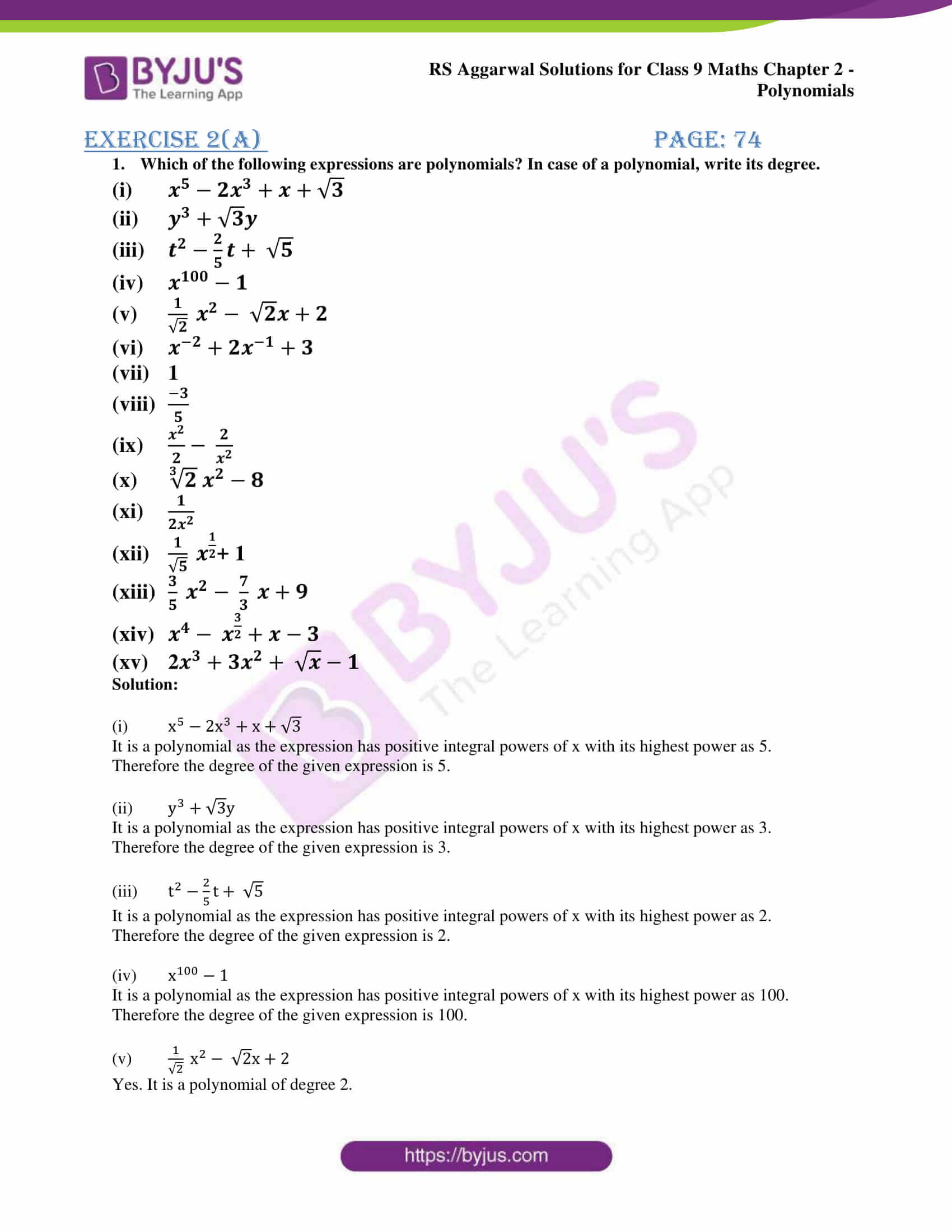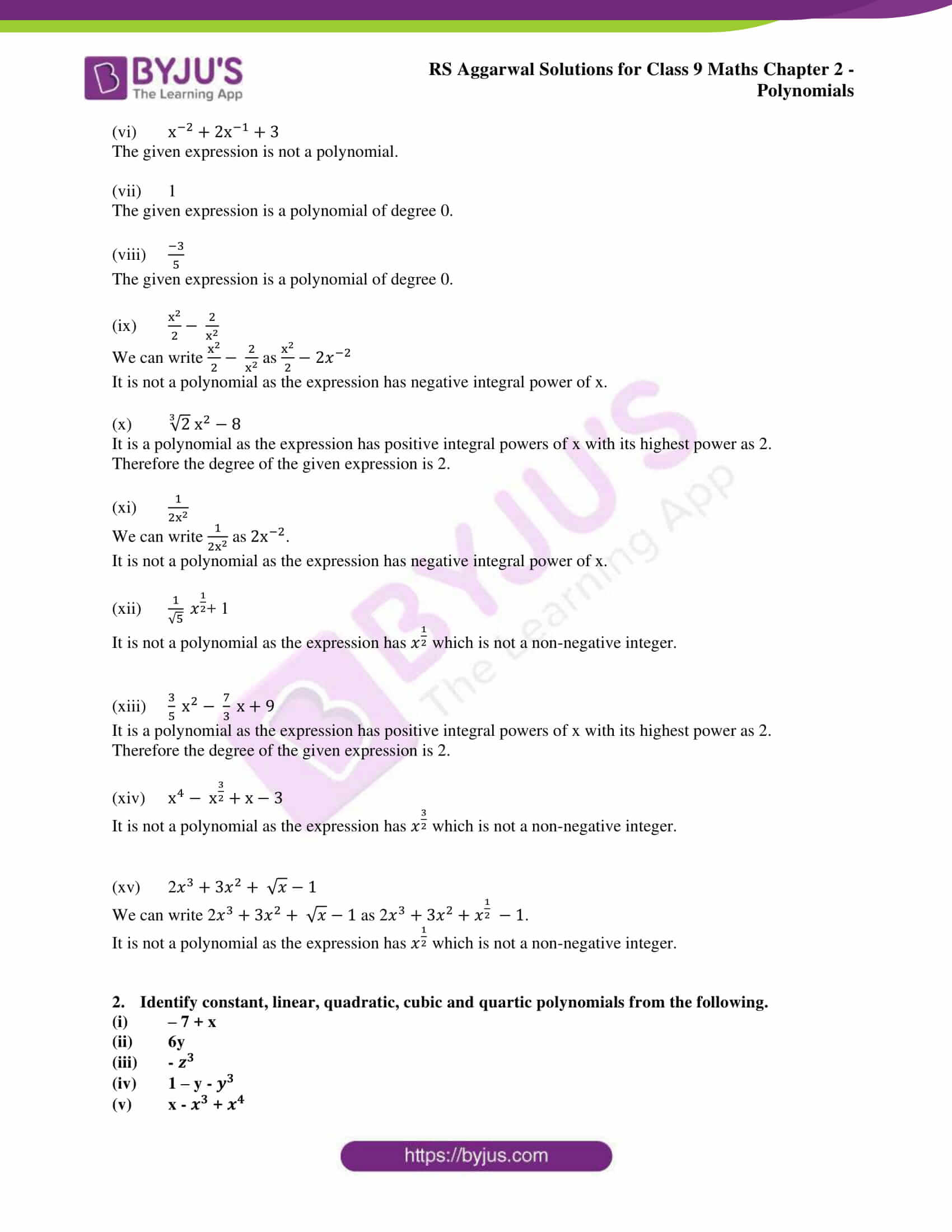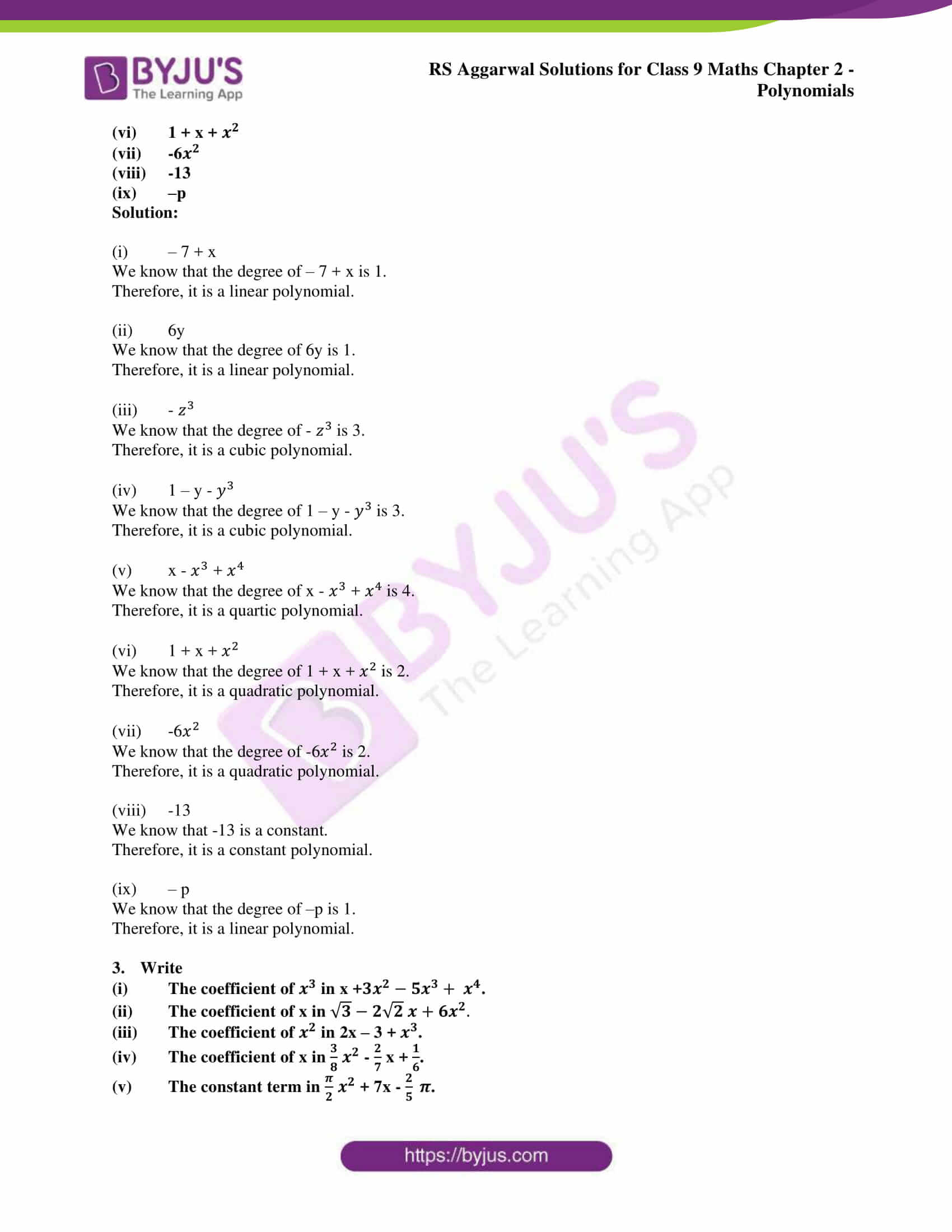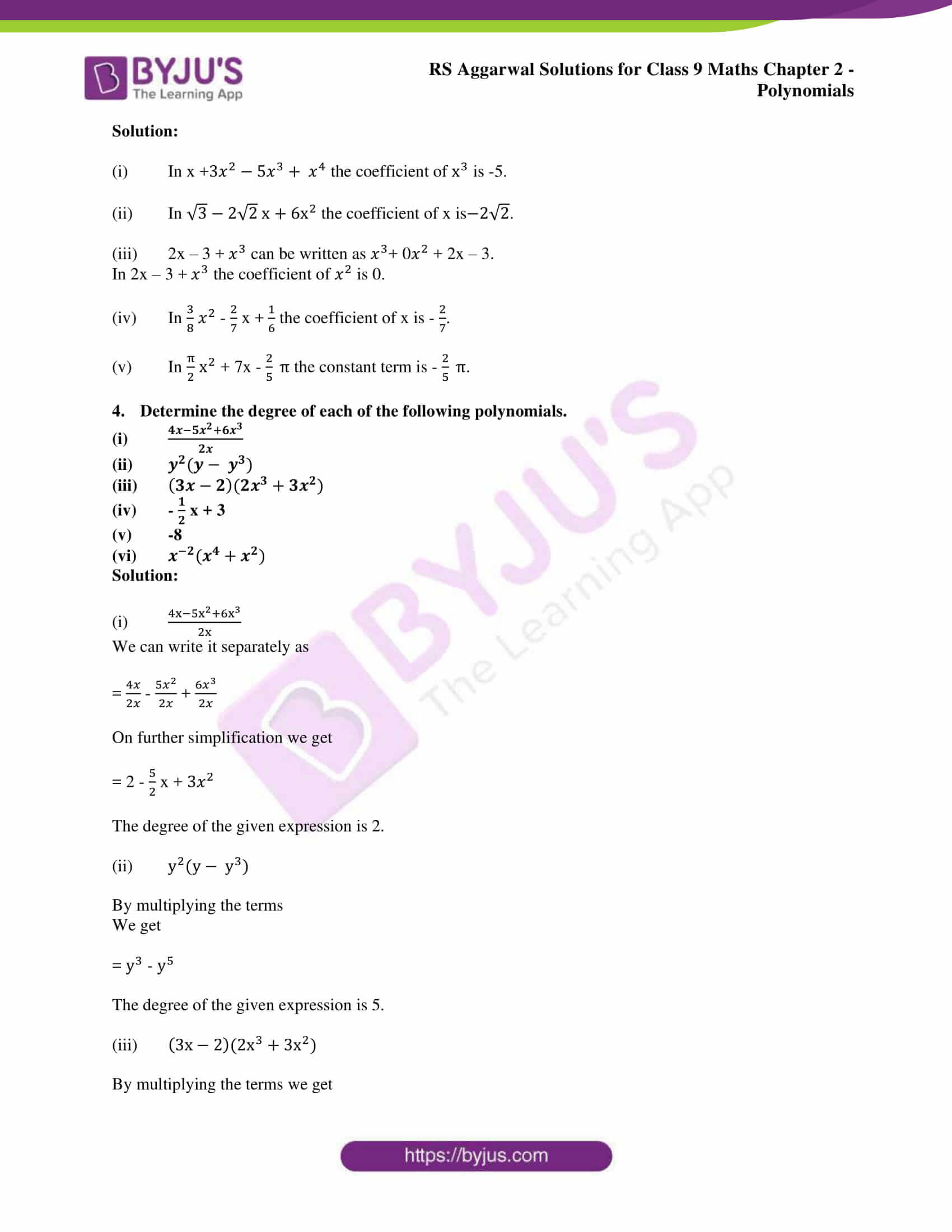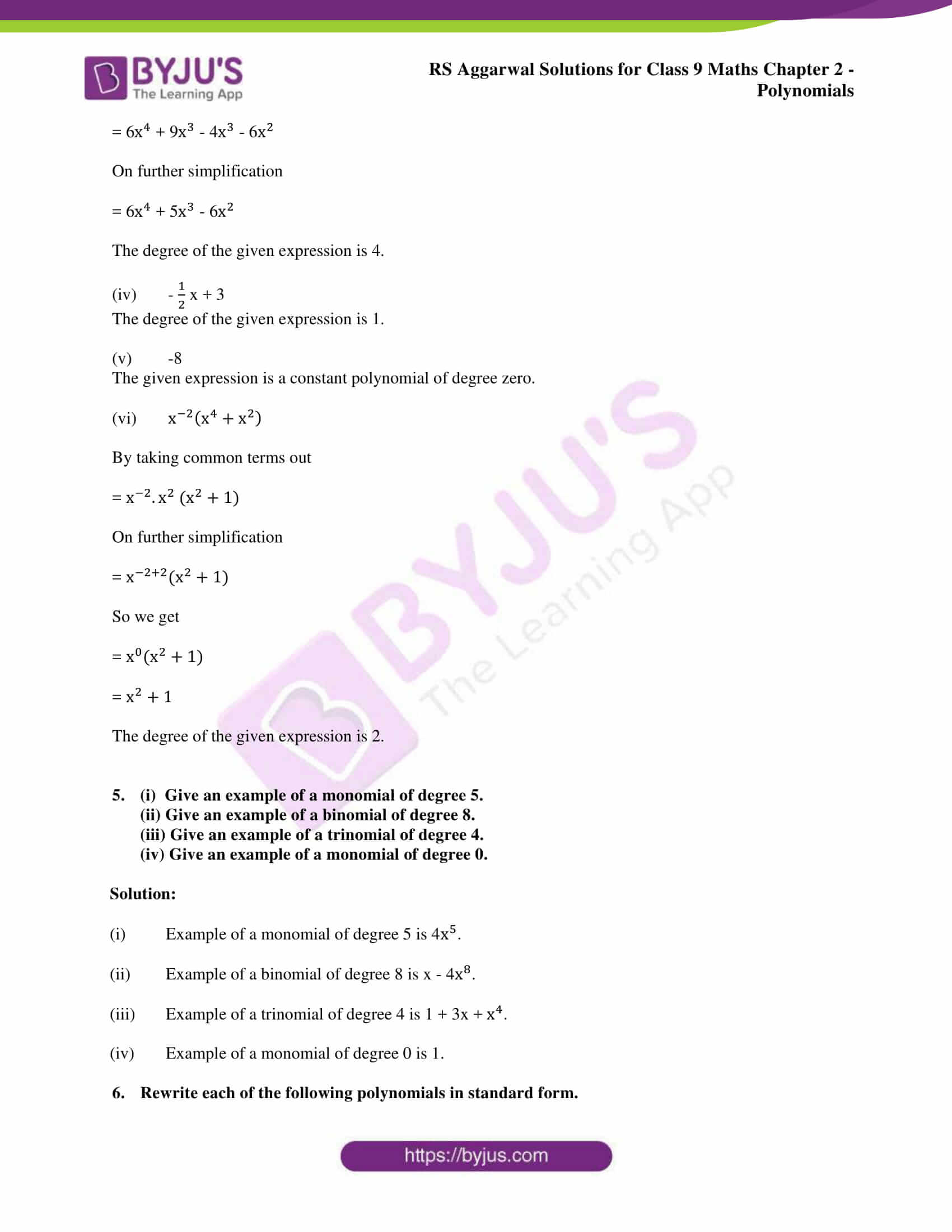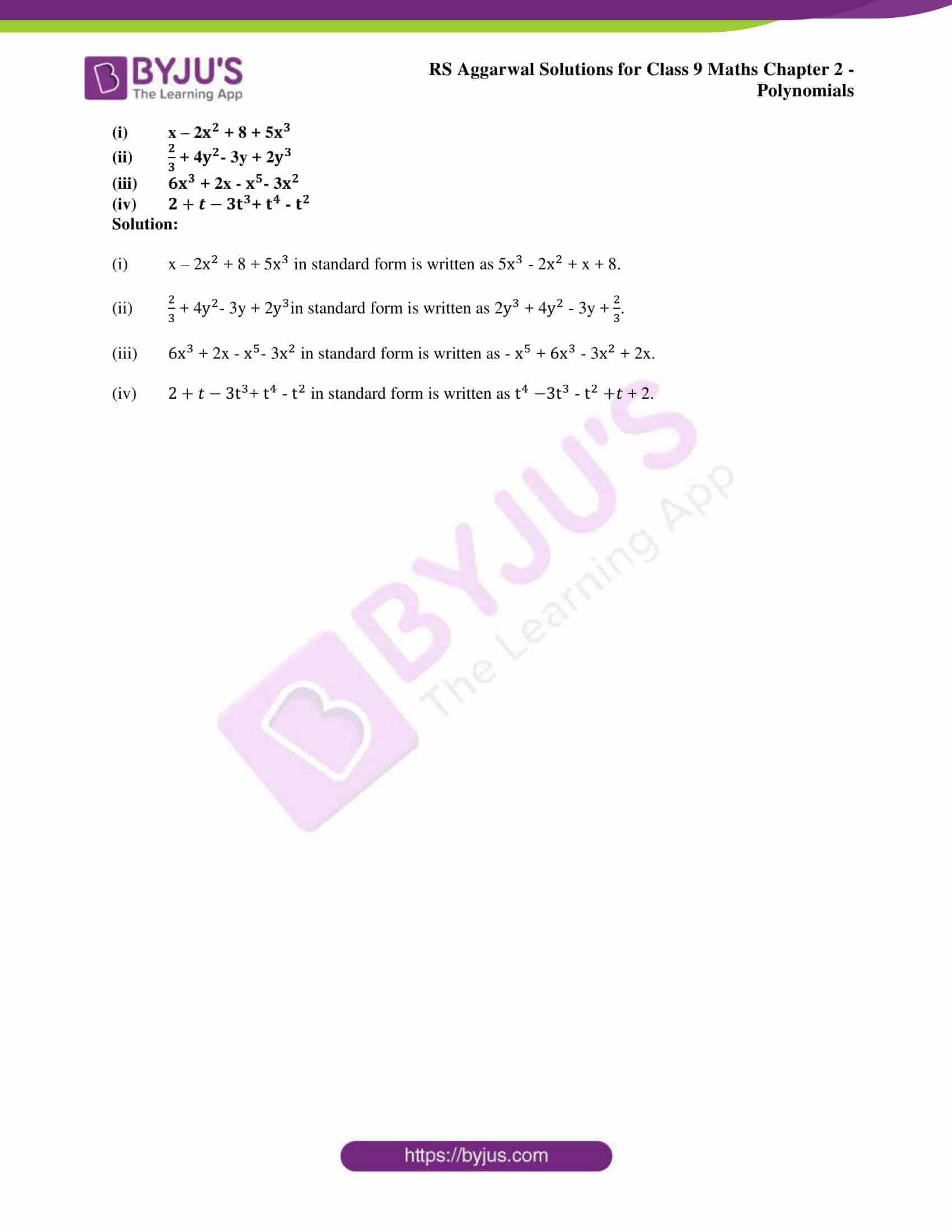### Access other exercise solutions of class 9 Maths Chapter 2: Polynomials

Exercise 2B Solutions 9 Questions

Exercise 2C Solutions 16 Questions

Exercise 2D Solutions 26 Questions

### RS Aggarwal Solutions Class 9 Maths Chapter 2 – Polynomials Exercise 2A

RS Aggarwal Solutions Class 9 Maths Chapter 2 Polynomials Exercise 2A has problems covering the introduction concepts of the chapter namely Polynomials in one variable, Polynomials of Various degrees and Number of terms in a Polynomial.

### Key features of RS Aggarwal Solutions for Class 9 Maths Chapter 2: Polynomials Exercise 2A

• The RS Aggarwal Solutions are prepared by subject experts who are highly skilled with vast experience in Mathematics.
• The solutions are solved in a manner which improves confidence among students to face the examination without fear.
• Students can make use of the PDF format during the final exam preparation as a revision material to understand the concepts.
• Long and difficult problems in the textbook can be solved using various methods from exam point of view.